Latest Banking jobs   »

# Quantitative Aptitude Quiz For IBPS Clerk Prelims 2022- 11th July

Directions (1-5): In each of these questions, two equations (I) and (II) are given. You have to solve both the equations and give answer
(a) if x > y                                       .
(b) if x ≥ y
(c) if x = y or no relation can be established between x and y.
(d) if y > x
(e) if y ≥ x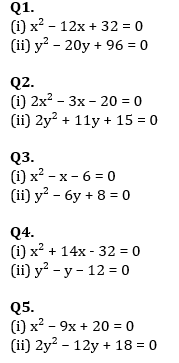(a) x > y                                                        .
(b) x ≥ y
(c) x < y
(d) x ≤ y
(e) x = y or no relation can be established between x and y.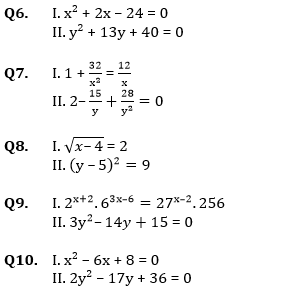Directions (11-15): In each of these questions, two equations (I) and (II) are given. You have to solve both the equations and give answer
(a) if x > y                                                                      .
(b) if x ≥ y
(c) if x = y or no relation can be established between x and y.
(d) if y > x                                                   .
(e) if y ≥ x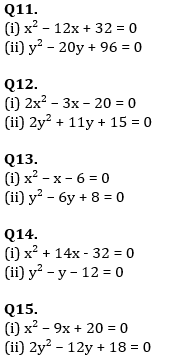Solutions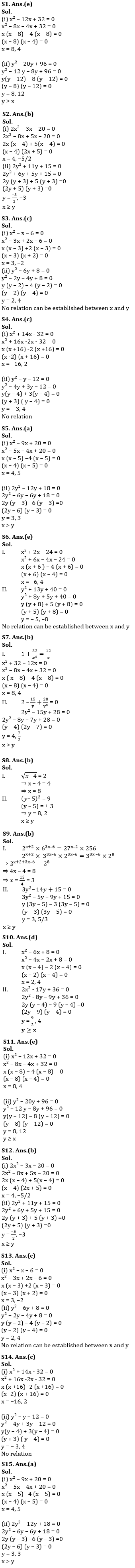#### Congratulations!Incorrect details? Fill the form again here

•Reasoning Ability Quiz For IBPS Clerk Pr...
•Reasoning Ability Quiz For IBPS Clerk Pr...
•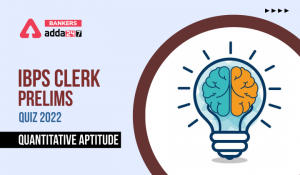Quantitative Aptitude Quiz For IBPS Cler...
•English Quizzes For IBPS Clerk Prelims 2...
•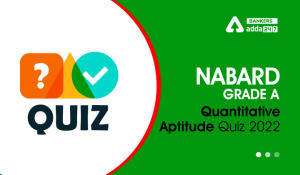Quantitative Aptitude Quiz For NABARD Gr...
•Reasoning Ability Quiz For IBPS Clerk Pr...